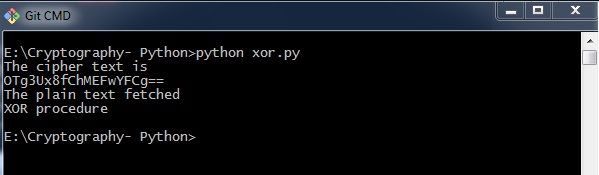# Cryptography with Python - XOR Process

In this chapter, let us understand the XOR process along with its coding in Python.

## Algorithm

XOR algorithm of encryption and decryption converts the plain text in the format ASCII bytes and uses XOR procedure to convert it to a specified byte. It offers the following advantages to its users −

• Fast computation
• No difference marked in left and right side
• Easy to understand and analyze

### Code

You can use the following piece of code to perform XOR process −

def xor_crypt_string(data, key = 'awesomepassword', encode = False, decode = False):
from itertools import izip, cycle
import base64

if decode:
data = base64.decodestring(data)
xored = ''.join(chr(ord(x) ^ ord(y)) for (x,y) in izip(data, cycle(key)))

if encode:
return base64.encodestring(xored).strip()
return xored
secret_data = "XOR procedure"

print("The cipher text is")
print xor_crypt_string(secret_data, encode = True)
print("The plain text fetched")
print xor_crypt_string(xor_crypt_string(secret_data, encode = True), decode = True)


### Output

The code for XOR process gives you the following output −### Explanation

• The function xor_crypt_string() includes a parameter to specify mode of encode and decode and also the string value.

• The basic functions are taken with base64 modules which follows the XOR procedure/ operation to encrypt or decrypt the plain text/ cipher text.

Note − XOR encryption is used to encrypt data and is hard to crack by brute-force method, that is by generating random encrypting keys to match with the correct cipher text.